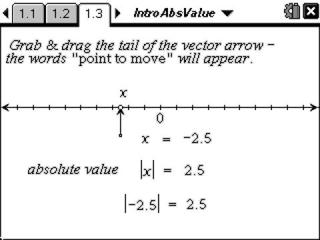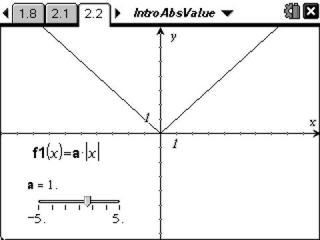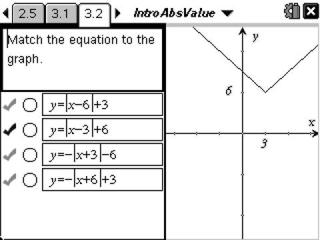# Activities

••• ##### Subject Area

• Math: Algebra II: Equations

• ##### Author9-12

45 Minutes

• ##### Device
• TI-Nspire™ Navigator™
• TI-Nspire™
• TI-Nspire™ CAS
• ##### Software

TI-Nspire™
TI-Nspire™ CAS

2.0

## Introduction to Absolute Value#### Activity Overview

In this activity students will explore the definition of absolute value using a number line, plot points to graph y = |x|, and use sliders to perform transformations with absolute value functions.

#### Key Steps

•In the first part of this activity, students will use a number line to understand the concept of absolute value as the distance from zero. Then they will enter positive and negative x values into a spreadsheet, which graphs the points (x, abs(x)).

•For the second part of the activity, students will investigate the effects of the variables a, c, and d on the graph of the function, y = a|x| and y = |x + d| + c.

•Students will check their understanding of Problem 2 by determining the equation of a given graph. These matching exercises are self-check.